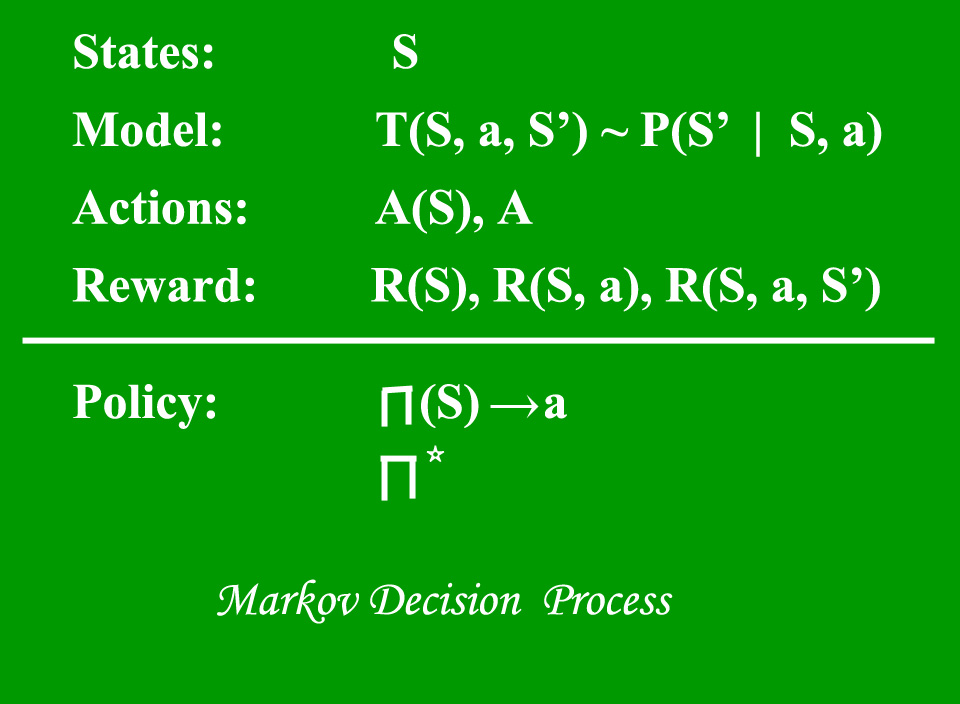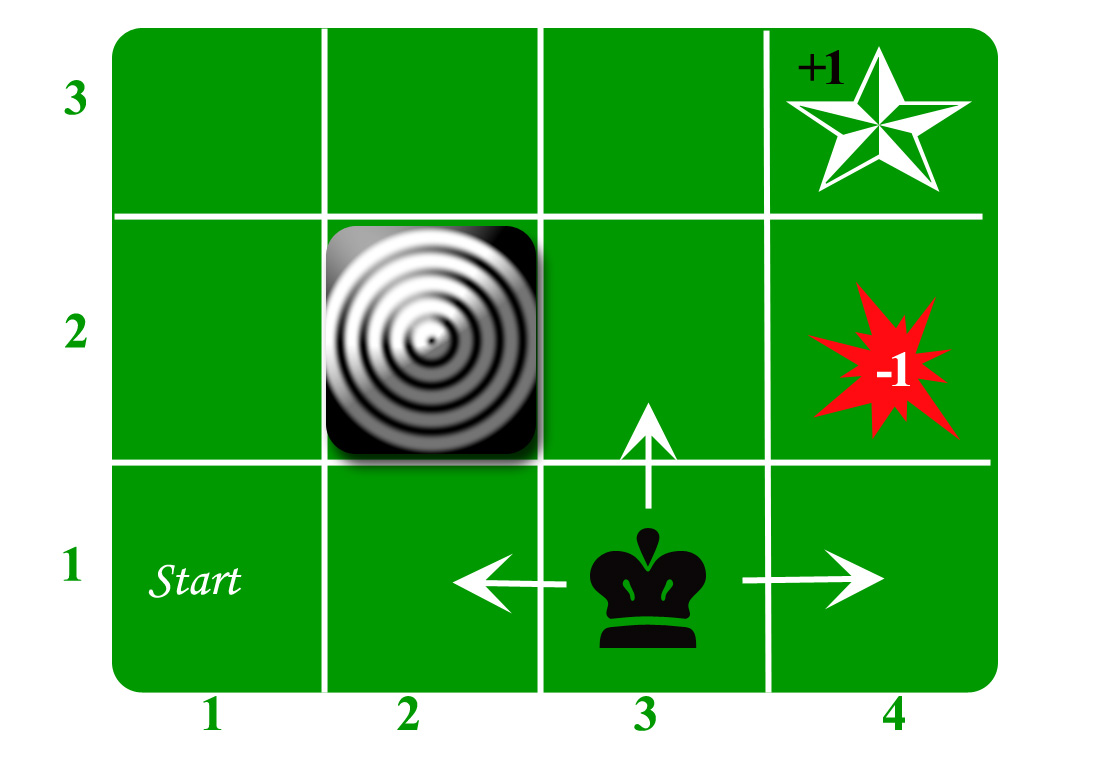# Markov Decision Process

• Difficulty Level : Medium
• Last Updated : 18 Nov, 2021

### Reinforcement Learning :

Reinforcement Learning is a type of Machine Learning. It allows machines and software agents to automatically determine the ideal behavior within a specific context, in order to maximize its performance. Simple reward feedback is required for the agent to learn its behavior; this is known as the reinforcement signal.

There are many different algorithms that tackle this issue. As a matter of fact, Reinforcement Learning is defined by a specific type of problem, and all its solutions are classed as Reinforcement Learning algorithms. In the problem, an agent is supposed to decide the best action to select based on his current state. When this step is repeated, the problem is known as a Markov Decision Process

A Markov Decision Process (MDP) model contains:

• A set of possible world states S.
• A set of Models.
• A set of possible actions A.
• A real-valued reward function R(s,a).
• A policy the solution of Markov Decision Process.### What is a State?

A State is a set of tokens that represent every state that the agent can be in.

### What is a Model?

A Model (sometimes called Transition Model) gives an action’s effect in a state. In particular, T(S, a, S’) defines a transition T where being in state S and taking an action ‘a’ takes us to state S’ (S and S’ may be the same). For stochastic actions (noisy, non-deterministic) we also define a probability P(S’|S,a) which represents the probability of reaching a state S’ if action ‘a’ is taken in state S. Note Markov property states that the effects of an action taken in a state depend only on that state and not on the prior history.

### What are Actions?

An Action A is a set of all possible actions. A(s) defines the set of actions that can be taken being in state S.

### What is a Reward?

A Reward is a real-valued reward function. R(s) indicates the reward for simply being in the state S. R(S,a) indicates the reward for being in a state S and taking an action ‘a’. R(S,a,S’) indicates the reward for being in a state S, taking an action ‘a’ and ending up in a state S’.

### What is a Policy?

A Policy is a solution to the Markov Decision Process. A policy is a mapping from S to a. It indicates the action ‘a’ to be taken while in state S.
Let us take the example of a grid world:An agent lives in the grid. The above example is a 3*4 grid. The grid has a START state(grid no 1,1). The purpose of the agent is to wander around the grid to finally reach the Blue Diamond (grid no 4,3). Under all circumstances, the agent should avoid the Fire grid (orange color, grid no 4,2). Also the grid no 2,2 is a blocked grid, it acts as a wall hence the agent cannot enter it.

The agent can take any one of these actions: UP, DOWN, LEFT, RIGHT

Walls block the agent path, i.e., if there is a wall in the direction the agent would have taken, the agent stays in the same place. So for example, if the agent says LEFT in the START grid he would stay put in the START grid.

First Aim: To find the shortest sequence getting from START to the Diamond. Two such sequences can be found:

• RIGHT RIGHT UP UPRIGHT
• UP UP RIGHT RIGHT RIGHT

Let us take the second one (UP UP RIGHT RIGHT RIGHT) for the subsequent discussion.
The move is now noisy. 80% of the time the intended action works correctly. 20% of the time the action agent takes causes it to move at right angles. For example, if the agent says UP the probability of going UP is 0.8 whereas the probability of going LEFT is 0.1, and the probability of going RIGHT is 0.1 (since LEFT and RIGHT are right angles to UP).

The agent receives rewards each time step:-

• Small reward each step (can be negative when can also be term as punishment, in the above example entering the Fire can have a reward of -1).
• Big rewards come at the end (good or bad).
• The goal is to Maximize the sum of rewards.
My Personal Notes arrow_drop_up
Recommended Articles
Page :